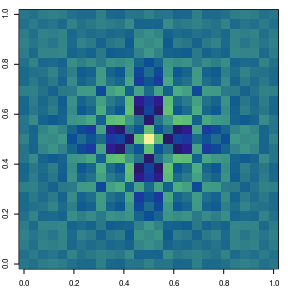# Beautiful colormaps for oceanography: cmocean

This package contains an R version of the cmocean color palettes for oceanography, developed originally for python by Kristen Thyng. The important detail of all the cmocean palettes is that they are all perceptually uniform.

Users interested in the the details of the original package development should consult the publication: Thyng, K. M., Greene, C. A., Hetland, R. D., Zimmerle, H. M., & DiMarco, S. F. (2016). True colors of oceanography. Oceanography, 29(3), 10.

# Examples:

The functions that return the colors are accessed using the cmocean() function, where the first argument is the name of the desired palette.

We can make a simple function to display the palette colors:

library(cmocean)
plot_cm <- function(name, n=256) {
z <- matrix(seq(0, 1, length.out=n))
image(z, col=cmocean(name)(n), axes=FALSE)
}

Below are examples of each of the cmocean palettes. Many more examples are provided at https://matplotlib.org/cmocean/

## palettes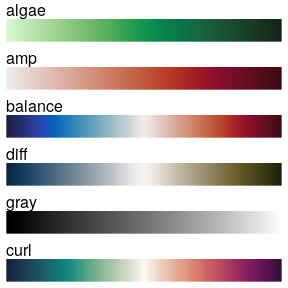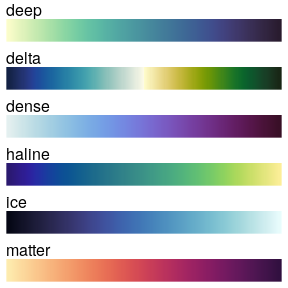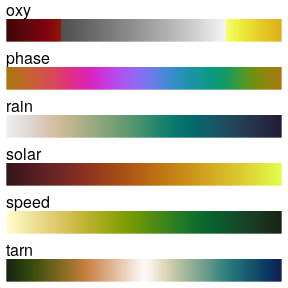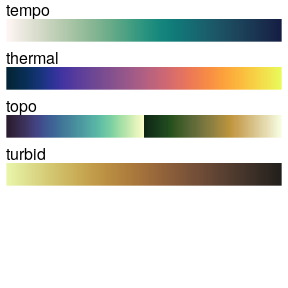## Data examples:

par(mar=c(2, 2, 1, 1), cex=0.5)
image(volcano, col=cmocean('thermal')(256))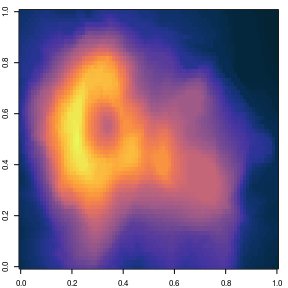par(mar=c(2, 2, 1, 1), cex=0.5)
x <- y <- seq(-4*pi, 4*pi, len = 27)
r <- sqrt(outer(x^2, y^2, "+"))
image(z = z <- cos(r^2)*exp(-r/6), col  = cmocean('haline')(256))